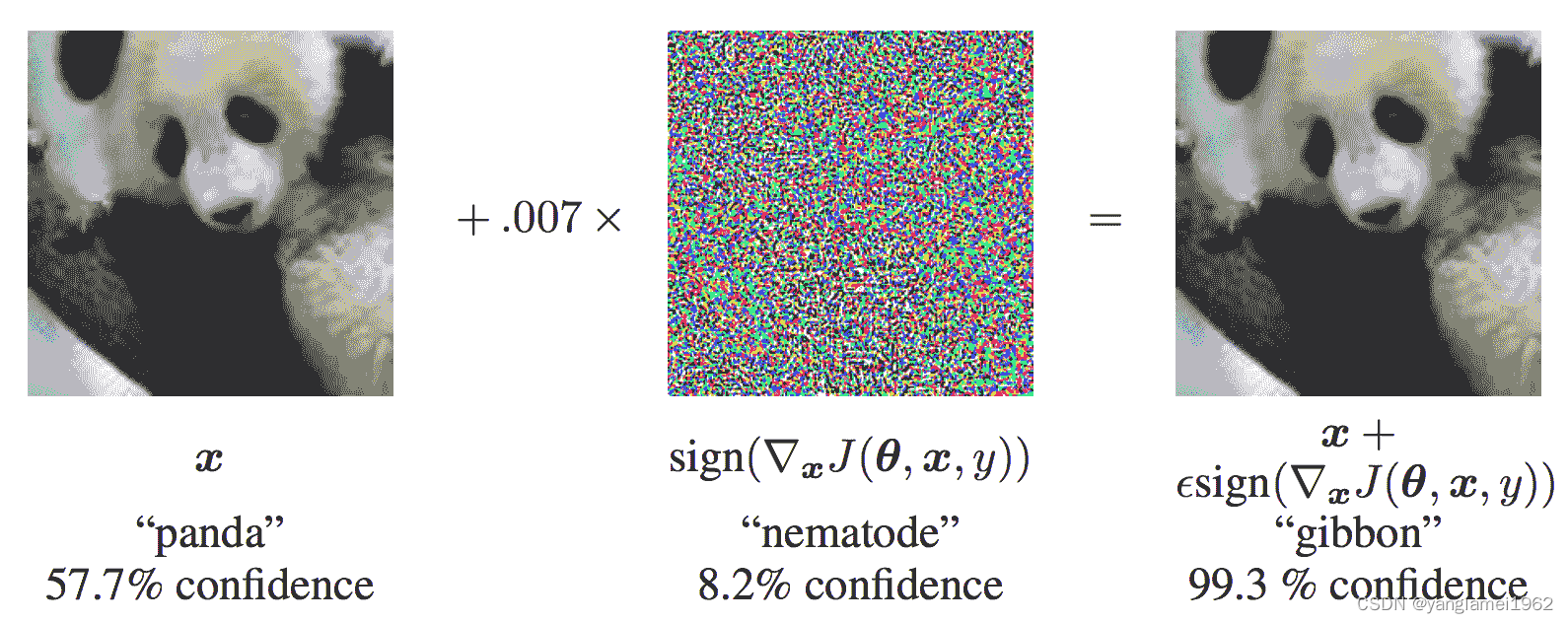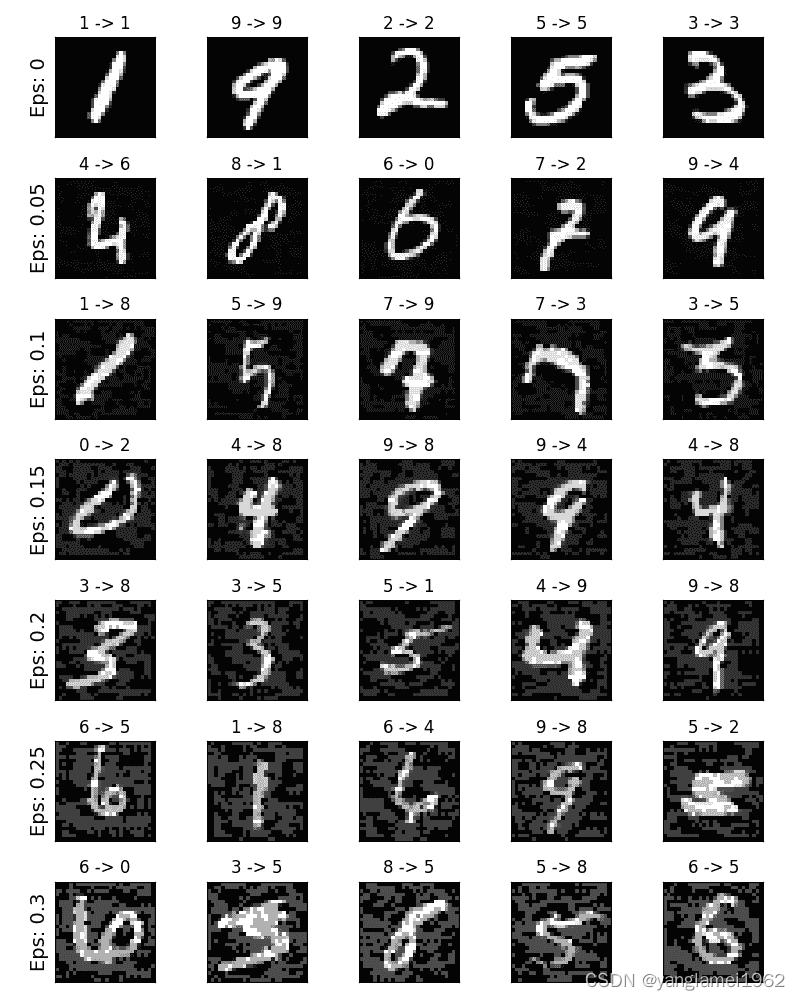# Adversarial Example Generation, Fast Gradient Sign Attack (FGSM) to Fool the MNIST Classifier

If you’re reading this, hopefully you can appreciate the effectiveness of some machine learning models. Research is constantly pushing ML models to be faster, more accurate and more efficient. However, an often overlooked aspect of designing and training models is security and robustness, especially in the face of adversaries looking to deceive the model.

This tutorial will increase your awareness of the security vulnerabilities of ML models and provide insight into the hot topic of adversarial machine learning. You may be surprised to learn that adding imperceptible perturbations to images can lead to completely different model performance. Since this is a tutorial, we’ll explore the topic with an example on an image classifier. Specifically, we will use one of the most popular attack methods, the Fast Gradient Sign Attack (FGSM), to fool the MNIST classifier.

## Threat model

For context, there are multiple types of adversarial attacks, each with different goals and assumptions. In general, however, the overall goal is to add the least amount of perturbation to the input data to induce the desired misclassification. There are several assumptions about the attacker’s knowledge, two of which are: white box and black box. White-box attacks assume that the attacker has complete knowledge and access to the model, including architecture, inputs, outputs, and weights. Black-box attacks assume that the attacker only has access to the input and output of the model and knows nothing about the underlying architecture or weights. There are also several types of destinations, including Misclassification and Source/Destination Misclassification. Misclassification means that the adversary only wants the output misclassification and does not care what the new classification is. Source/Target Misclassification means that an adversary wants to change an image that originally belonged to a certain source category in order to classify it as a certain target category.

In this case, the FGSM attack is a white box attack targeting misclassification. With this background information, we can now discuss the attack in detail.

Before diving into the code, let’s look at the famous FGSM Pandas example and extract some symbols.From the figure, `x` is the original input image correctly classified as “Pandas”, `y` is the output of `x`, `θ` code> represents the model parameters, and `J(θ, x, y)` is the loss used to train the network. The attack backpropagates the gradients back to the input data to compute `?[x] J(θ, x, y)`. Then, it takes a small step (`ε` or `0.007` in the picture) along the direction (i.e. `?[x] J(θ)`) Adjust the input data, `(x, y)`, which will maximize the loss. Then, the target network misclassified them as “Gibbons” when the target images were still clearly “Pandas”.

Hopefully the motivation for this tutorial is clear, so let’s jump into the implementation.

```from __future__ import print_function
import torch
import torch.nn as nn
import torch.nn.functional as F
import torch.optim as optim
from torchvision import datasets, transforms
import numpy as np
import matplotlib.pyplot as plt

```

## Implementation

In this section, we discuss the input parameters for this tutorial, define the model under attack, then write the attack code and run some tests.

### Enter

This tutorial has only three inputs, defined as follows:

• `epsilons` – A list of `ε` values to use for the run. It is important to keep 0 in the list because it represents the model performance on the original test set. Similarly, intuitively, we expect that the larger the `ε`, the more obvious the perturbation, but from the perspective of reducing the accuracy of the model, the attack is more effective. Since the data range here is `[0,1]`, the value of `ε` cannot exceed 1.
• `pretrained_model` – Path to the MNIST model trained using `pytorch/examples/mnist`. For simplicity, download the pretrained model here.
• `use_cuda` – Boolean flag to use CUDA if required and available. Note that a GPU with CUDA is not important in this tutorial as the CPU won’t spend much time.
```epsilons = [0, .05, .1, .15, .2, .25, .3]
pretrained_model = "data/lenet_mnist_model.pth"
use_cuda=True

```

### Model under attack

As mentioned earlier, the model under attack is the same as the MNIST model in `pytorch/examples/mnist`. You can train and save your own MNIST model, or download and use the provided model. The network definition and test data loader here have been copied from the MNIST example. The purpose of this section is to define the model and data loader, then initialize the model and load the pretrained weights.

```# LeNet Model definition
class Net(nn.Module):
def __init__(self):
super(Net, self).__init__()
self.conv1 = nn.Conv2d(1, 10, kernel_size=5)
self.conv2 = nn.Conv2d(10, 20, kernel_size=5)
self.conv2_drop = nn.Dropout2d()
self.fc1 = nn.Linear(320, 50)
self.fc2 = nn.Linear(50, 10)

def forward(self, x):
x = F.relu(F.max_pool2d(self.conv1(x), 2))
x = F.relu(F.max_pool2d(self.conv2_drop(self.conv2(x)), 2))
x = x.view(-1, 320)
x = F.relu(self.fc1(x))
x = F. dropout(x, training=self. training)
x = self.fc2(x)
return F.log_softmax(x, dim=1)

# MNIST Test dataset and dataloader declaration
transforms.ToTensor(),
])),
batch_size=1, shuffle=True)

# Define what device we are using
print("CUDA Available: ",torch.cuda.is_available())
device = torch.device("cuda" if (use_cuda and torch.cuda.is_available()) else "cpu")

# Initialize the network
model = Net().to(device)

# Set the model in evaluation mode. In this case this is for the Dropout layers
model.eval()

```

out:

```Downloading http://yann.lecun.com/exdb/mnist/train-images-idx3-ubyte.gz to ../data/MNIST/raw/train-images-idx3-ubyte.gz
Extracting ../data/MNIST/raw/train-images-idx3-ubyte.gz to ../data/MNIST/raw
Extracting ../data/MNIST/raw/train-labels-idx1-ubyte.gz to ../data/MNIST/raw
Extracting ../data/MNIST/raw/t10k-images-idx3-ubyte.gz to ../data/MNIST/raw
Extracting ../data/MNIST/raw/t10k-labels-idx1-ubyte.gz to ../data/MNIST/raw
Processing...
Done!
CUDA Available: True

```

### FGSM attack

We can now define functions that create adversarial examples by perturbing the original input. The `fgsm_attack` function accepts three inputs, `image` is the original clean image (`x`), `epsilon` is the pixel-level perturbation Quantity `ε`, `data_grad` is the gradient of the input image loss (`?[x] J(θ, x, y)`). The function then creates the perturbed image as

[External link picture transfer failed, the source site may have an anti-leeching mechanism, it is recommended to save the picture and upload it directly (img-huc923wt-1684650061780)(img/tex21-1.gif)]

Finally, to maintain the original range of the data, the perturbed image is cropped to the range `[0,1]`.

```# FGSM attack code
# Collect the element-wise sign of the data gradient
# Create the perturbed image by adjusting each pixel of the input image
# Adding clipping to maintain [0,1] range
perturbed_image = torch.clamp(perturbed_image, 0, 1)
# Return the perturbed image
return perturbed_image

```

### Test function

Finally, the main results of this tutorial come from the `test` function. Each call to this test function performs the full test step on the MNIST test set and reports the final accuracy. Note, however, that this function also requires an `epsilon` input. This is because the `test` function reports the accuracy of the attack model from the adversary with strength `ε`. More specifically, for each sample in the test set, the function computes the gradient of the loss on the input data `data_grad` and uses `fgsm_attack` to create a perturbed image `perturbed_data` code>, and then check whether the perturbed examples are adversarial. In addition to testing the accuracy of the model, this function also saves and returns some successful adversarial examples for later visualization.

```def test( model, device, test_loader, epsilon ):

# Accuracy counter
correct = 0

# Loop over all examples in test set

# Send the data and label to the device
data, target = data.to(device), target.to(device)

# Set requires_grad attribute of tensor. Important for Attack

# Forward pass the data through the model
output = model(data)
init_pred = output.max(1, keepdim=True) # get the index of the max log-probability

# If the initial prediction is wrong, don't bother attacking, just move on
if init_pred.item() != target.item():
continue

# Calculate the loss
loss = F.nll_loss(output, target)

# Calculate gradients of model in backward pass
loss. backward()

# Call FGSM Attack

# Re-classify the perturbed image
output = model(perturbed_data)

# Check for success
final_pred = output.max(1, keepdim=True) # get the index of the max log-probability
if final_pred.item() == target.item():
correct += 1
# Special case for saving 0 epsilon examples
if (epsilon == 0) and (len(adv_examples) < 5):
else:
# Save some adv examples for visualization later

# Calculate final accuracy for this epsilon
print("Epsilon: {}\tTest Accuracy = {} / {} = {}".format(epsilon, correct, len(test_loader), final_acc))

# Return the accuracy and an adversarial example

```

### Run attack

The final part of the implementation is actually running the attack. Here we run the full test step for each value of `ε` in the `epsilon` input. For each `ε`, we also save the final accuracy and plot some successful adversarial examples in the next section. Note how the accuracy of the print decreases as the value of ε increases. In addition, please note that `ε = 0` means the original test accuracy, not under attack.

```accuracies = []
examples = []

# Run test for each epsilon
for eps in epsilons:
acc, ex = test(model, device, test_loader, eps)
accuracies. append(acc)
examples.append(ex)

```

out:

```Epsilon: 0 Test Accuracy = 9810 / 10000 = 0.981
Epsilon: 0.05 Test Accuracy = 9426 / 10000 = 0.9426
Epsilon: 0.1 Test Accuracy = 8510 / 10000 = 0.851
Epsilon: 0.15 Test Accuracy = 6826 / 10000 = 0.6826
Epsilon: 0.2 Test Accuracy = 4301 / 10000 = 0.4301
Epsilon: 0.25 Test Accuracy = 2082 / 10000 = 0.2082
Epsilon: 0.3 Test Accuracy = 869 / 10000 = 0.0869

```

## Results

### Accuracy and `ε`

The first result is accuracy versus the `ε` curve. As mentioned earlier, we expect a decrease in test accuracy as `ε` increases. This is because a larger ε means we are taking a larger step towards maximizing the loss. Note that even though the `ε` values are spaced linearly, the trend in the curve is not linear. For example, `ε = 0.05` is only about 4% less accurate than `ε = 0`, but `ε = 0.2` is less accurate than ` ε = 0.15`. Also, notice that the accuracy of the model is between `ε = 0.25` and `ε = 0.3` to achieve the random accuracy of a 10-class classifier.

```plt.figure(figsize=(5,5))
plt.plot(epsilons, accuracies, "*-")
plt.yticks(np.arange(0, 1.1, step=0.1))
plt. xticks(np. arange(0, .35, step=0.05))
plt. title("Accuracy vs Epsilon")
plt.xlabel("Epsilon")
plt.ylabel("Accuracy")
plt. show()

```Remember the idea of no free lunch? In this case, as `ε` increases, the test accuracy decreases, but the perturbation becomes more perceptible. In practice, there is a trade-off between accuracy degradation and perceptibility that an attacker must consider. Here we show some examples of successful adversarial examples for each value of `ε`. Each row of the plot shows a different `ε` value. The first row is the `ε = 0` examples, which represent the original “clean” images without distractions. The title of each image says “Original Classification -> Adversarial Classification”. Note that the perturbation starts to become noticeable at `ε = 0.15` and becomes very noticeable at `ε = 0.3`. However, in all cases, humans were able to identify the correct class despite the added noise.

```# Plot several examples of adversarial samples at each epsilon
cnt = 0
plt.figure(figsize=(8,10))
for i in range(len(epsilons)):
for j in range(len(examples[i])):
cnt + = 1
plt.subplot(len(epsilons),len(examples),cnt)
plt. xticks ([], [])
plt. yticks ([], [])
if j == 0:
plt.ylabel("Eps: {}".format(epsilons[i]), fontsize=14)